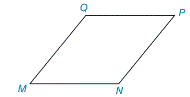Chapter 8.1, Problem 6E### Elementary Geometry for College St...

6th Edition
Daniel C. Alexander + 1 other
ISBN: 9781285195698

#### Solutions

Chapter
Section### Elementary Geometry for College St...

6th Edition
Daniel C. Alexander + 1 other
ISBN: 9781285195698
Textbook Problem
1 views

# When the diagonals of rhombus MNPQ are drawn, how do the areas of the four resulting smaller triangles compare to each other and to the area of the given rhombus?Exercise 4–6

To determine

To compare:

The area of the four resulting smaller triangle to each other and to the area of the given rhombus.

Explanation

Given,

If MNPQ is a rhombus

Rhombus has four equal sides and its diagonals bisect each other at right angles.

Here,

The rhombus of diagonal d1=MP

The rhombus of diagonal d2=NQ

From the diagram,

Here, ON=OQ=d22 and OP=OM=d12

ΔNOM,ΔPON,ΔQOP and ΔMOQ are the right triangles in the rhombus.

Thus, the area of rhombus is equal to the sum of the area of right triangles ΔNOM(A1),ΔPON(A2),ΔQOP(A3) and ΔMOQ(A4)

The area of rhombus (A)=A1+A2+A3+A4

For the right ΔNOM,

The area of the right ΔNOM(A1)=12ab unit2

Here, ON=a=d22 and OM=b=d12

A1=12d22d12

=d2d18

A1=d1d28

For the right ΔPON,

Area of the right ΔPON(A2)=12ab

Here, ON=a=d22 and OP=b=d12

A2=12d22d12

A2=d2d18

A2=d1d28

For the right ΔQOP,

Area of the right ΔQOP(A3)=12ab

OQ=a=d22 and OP=b=d12

A3=12d22d12

=d

### Still sussing out bartleby?

Check out a sample textbook solution.

See a sample solution

#### The Solution to Your Study Problems

Bartleby provides explanations to thousands of textbook problems written by our experts, many with advanced degrees!

Get Started

#### Differentiate. y=ep(p+pp)

Single Variable Calculus: Early Transcendentals, Volume I

#### In Exercise 11-14, factor the expression. x2+5x+6

Calculus: An Applied Approach (MindTap Course List)

#### In Exercises 49-62, find the indicated limit, if it exists. 59. limx1x1x1

Applied Calculus for the Managerial, Life, and Social Sciences: A Brief Approach

#### For

Study Guide for Stewart's Multivariable Calculus, 8th